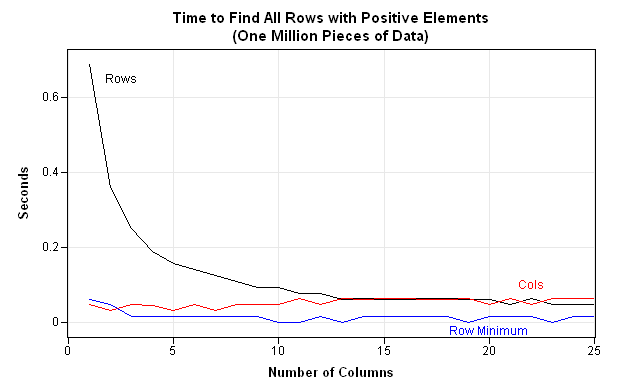In a previous post, I discussed how to use the LOC function to eliminate loops over observations. Dale McLerran chimed in to remind me that another way to improve efficiency is to use subscript reduction operators.

I ended my previous post by issuing a challenge: can you write an efficient module that finds the rows in a matrix for which all elements are positive?

The submissions I received basically fall into three categories: loop over rows, loop over columns, or use the subscript reduction operators to eliminate the loop. Let's time each algorithm and plot the relative performance as the number of columns varies.

### Looping over Rows

This will be the slowest method when there are millions of rows and few columns. However, as the number of columns increases, this method becomes competitive with the others. The following module is a typical implementation:

```proc iml; /** test for positive entries: loop over rows **/ start TestAllVarsPosByRows(x); b = j(nrow(x),1); do i = 1 to nrow(x); b[i] = all(x[i,] > 0); end; return( loc(b) ); finish;```

### Looping over Columns

This will be fast provided that there are many more rows than columns. The following module is typical:

```/** test for positive entries: loop over columns **/ start TestAllVarsPosByCols(x); b = (x[,1] > 0); /** initialize vector of 0's and 1's **/ do j = 2 to ncol(x); b = b & (x[,j] > 0); /** logical AND operator **/ end; return( loc(b) ); finish;```

### Subscript Reduction Operators

For the problem I posed, you can use the "minimum value" subscript reduction operator to eliminate all loops. Although you cannot use this trick every time that you want to find the rows that satisfy a condition, you should use subscript reduction operators when you can. Dale's implementation is as follows:

```/** no loop - use subscript reduction operators (Dale McLerran) **/ start TestAllVarsPosBySubRed(x); return( loc(x[ ,>< ] > 0) ); finish;```

### Comparing the Performance of the Algorithms

The performance of an algorithm depends on the size of the data that is processed. If you fix the size of the data at, say, one million numbers, the algorithms might depend on the number of rows and columns.

The following program generates one million random numbers from the standard normal distribution. These numbers are reshaped into matrices with 1, 2, 3, ..., 25 columns, and the time required for each module to run is recorded.

```y = j(1e6,1); call randseed(12345); call randgen(y, "Normal"); /** generate random numbers **/   p = 1:25; n = round(1e6 / p); results = j(ncol(n),5); do i = 1 to ncol(n); x = shape(y, n[i], p[i]); /** reshape data **/ /** time each algorithm **/ t0 = time(); r = TestAllVarsPosByRows(x); tRows = time()-t0; t0 = time(); c = TestAllVarsPosByCols(x); tCols = time()-t0; t0 = time(); s = TestAllVarsPosBySubRed(x); tSubs = time()-t0; results[i,] = n[i] || p[i] || tRows || tCols || tSubs; end;```### Interpreting the Results

The graph (which is created in SAS/IML Studio) shows the following:

• The algorithm that loops over rows is substantially slower than the other algorithms when there are fewer than 10 columns.
• For more than 10 columns, the row- and column-wise algorithms are comparable. Each algorithm processes the one million numbers in about 0.1 seconds.
• The subscript reduction algorithm processes the data almost instantly for all shapes of matrices.

The lesson is that your programs will run faster if you can reduce the use of loops. (This is not unique to SAS/IML programs, but is true of all high-level languages such as MATLAB and R.) In the SAS/IML language, both the LOC function and subscript reduction operators help you to eliminate loops in your program.

ShareDistinguished Researcher in Computational Statistics

Rick Wicklin, PhD, is a distinguished researcher in computational statistics at SAS and is a principal developer of SAS/IML software. His areas of expertise include computational statistics, simulation, statistical graphics, and modern methods in statistical data analysis. Rick is author of the books Statistical Programming with SAS/IML Software and Simulating Data with SAS.

1.2.